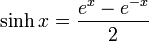# Hyperbolic sine function

Jump to: navigation, search
This article is about a particular function from a subset of the real numbers to the real numbers. Information about the function, including its domain, range, and key data relating to graphing, differentiation, and integration, is presented in the article.
View a complete list of particular functions on this wiki

## Definition

The hyperbolic sine function, denoted$\sinh$, is defined as follows:$\sinh x = \frac{e^x - e^{-x}}{2}$

Here,$e^x$ denotes the exponential function.

More abstractly, the hyperbolic sine function can be defined as the odd part of the exponential function.